# How to use Subtraction in Excel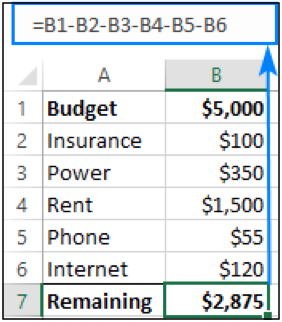In this article, we will learn how to apply Subtraction in Excel.

Scenario:

Subtraction is one of the four basic arithmetic operations, and every primary school pupil knows that to subtract one number from another you use the minus sign. This good old method works in Excel too. What kind of things can you subtract in your worksheets? Just any things which excel understands as numbers: such as numbers, percentages, days, months, hours, minutes and seconds.

Subtraction formula in Excel (minus formula)

You can even subtract matrices, text strings and lists. Now, let's take a look at how you can do all this.

Manual Subtract in Excel

Subtract cells in Excel

Subtract columns

Subtract percentage

Subtracting dates

Subtracting times

Matrix subtraction

Let's learn each of the above mentioned methods taking one by one to understand how subtraction works in excel.

Example :

All of these might be confusing to understand. Let's understand how to use the function using an example. Here To perform a simple subtraction operation, you use the minus sign (-).

The basic Excel subtraction formula is as simple as this:

=number1-number2

For example, to subtract 10 from 100, write the below equation and get 90 as the result:

=100-10

To enter the formula in your worksheet, do the following steps:

In a cell where you want the result to appear, type the equality sign (=).

Type the first number followed by the minus sign followed by the second number.

Complete the formula by pressing the Enter key.

Like in math, you can perform more than one arithmetic operation within a single formula.

For example, to subtract a few numbers from 100, type all those numbers separated by a minus sign:

=100-10-20-30

To indicate which part of the formula should be calculated first, use parentheses. For example:

=(100-10)/(80-20)

The screenshot below shows a few more formulas to subtract numbers in Excel: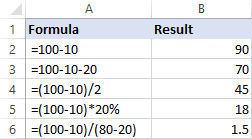## How to subtract cells in Excel

To subtract one cell from another, you also use the minus formula but supply cell references instead of actual numbers:

=cell_1 - cell_2

For example, to subtract the number in B2 from the number in A2, use this formula:

=A2-B2

You do not necessarily have to type cell references manually, you can quickly add them to the formula by selecting the corresponding cells. Here's how:

In the cell where you want to output the difference, type the equals sign (=) to begin your formula.

Click on the cell containing a minuend (a number from which another number is to be subtracted). Its reference will be added to the formula automatically (A2).

Type a minus sign (-).

Click on the cell containing a subtrahend (a number to be subtracted) to add its reference to the formula (B2).

Press the Enter key to complete your formula.

And you will have a result similar to this: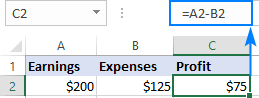## How to subtract multiple cells from one cell in Excel

To subtract multiple cells from the same cell, you can use any of the following methods.

#### Method 1. Minus sign

Simply type several cell references separated by a minus sign like we did when subtracting multiple numbers.

For example, to subtract cells B2:B6 from B1, construct a formula in this way:

=B1-B2-B3-B4-B5-B6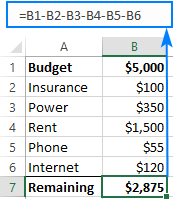#### Method 2. SUM function

To make your formula more compact, add up the subtrahends (B2:B6) using the SUM function, and then subtract the sum from the minuend (B1):

=B1-SUM(B2:B6)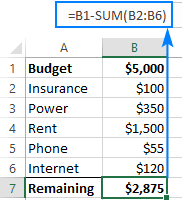#### Method 3. Sum negative numbers

As you may remember from a math course, subtracting a negative number is the same as adding it. So, make all the numbers you want to subtract negative (for this, simply type a minus sign before a number), and then use the SUM function to add up the negative numbers:

=SUM(B1:B6)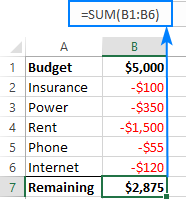## How to subtract columns in Excel

To subtract 2 columns row-by-row, write a minus formula for the topmost cell, and then drag the fill handle or double-click the plus sign to copy the formula to the entire column.

As an example, let's subtract numbers in column C from the numbers in column B, beginning with row 2:

=B2-C2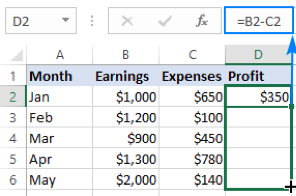Due to the use of relative cell references, the formula will adjust properly for each row:## Subtract the same number from a column of numbers

To subtract one number from a range of cells, enter that number in some cell (F1 in this example), and subtract cell F1 from the first cell in the range:

=B2-\$F\$1

The key point is to lock the reference for the cell to be subtracted with the \$ sign. This creates an absolute cell reference that does not change no matter where the formula is copied. The first reference (B2) is not locked, so it changes for each row.

As the result, in cell C3 you will have the formula =B3-\$F\$1; in cell C4 the formula will change to =B4-\$F\$1, and so on: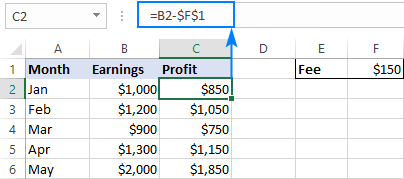If the design of your worksheet does not allow for an extra cell to accommodate the number to be subtracted, nothing prevents you from hardcoding it directly in the formula:

=B2-150

## How to subtract percentage in Excel

If you want to simply subtract one percentage from another, the already familiar minus formula will work a treat. For example:

=100%-30%

Or, you can enter the percentages in individual cells and subtract those cells:

=A2-B2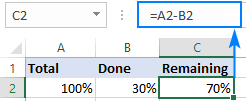If you wish to subtract percentage from a number, i.e. decrease number by percentage, then use this formula:

=Number * (1 - %)

For example, here's how you can reduce the number in A2 by 30%:

=A2*(1-30%)

Or you can enter the percentage in an individual cell (say, B2) and refer to that cell by using an absolute reference:

=A2*(1-\$B\$2)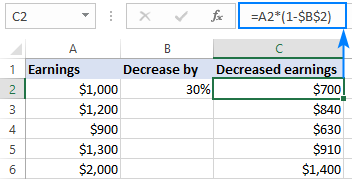## How to subtract dates in Excel

The easiest way to subtract dates in Excel is to enter them in individual cells, and subtract one cell from the other:

=End_date - Start_date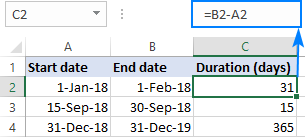You can also supply dates directly in your formula with the help of the DATE or DATEVALUE function. For example:

=DATE(2018,2,1)-DATE(2018,1,1)

=DATEVALUE("2/1/2018")-DATEVALUE("1/1/2018")

How to add and subtract dates in Excel

How to calculate days between dates in Excel

## How to subtract time in Excel

The formula for subtracting time in Excel is built in a similar way:

=End_time-Start_time

For example, to get the difference between the times in A2 and B2, use this formula:

=A2-B2

For the result to display correctly, be sure to apply the Time format to the formula cell: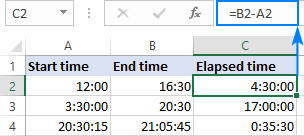You can achieve the same result by supplying the time values directly in the formula. For Excel to understand the times correctly, use the TIMEVALUE function:

=TIMEVALUE("4:30 PM")-TIMEVALUE("12:00 PM")

How to calculate time in Excel

How to add & subtract time to show over 24 hours, 60 minutes, 60 seconds

## How to do matrix subtraction in Excel

Suppose you have two sets of values (matrices) and you want to subtract the corresponding elements of the sets like shown in the screenshot below: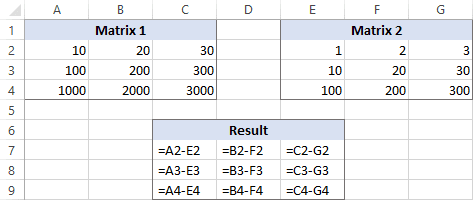Here's how you can do this with a single formula:

Select a range of empty cells that has the same number of rows and columns as your matrices.

In the selected range or in the formula bar, type the matrix subtraction formula:
=(A2:C4)-(E2:G4) , Now press Ctrl + Shift + Enter to make it an array formula.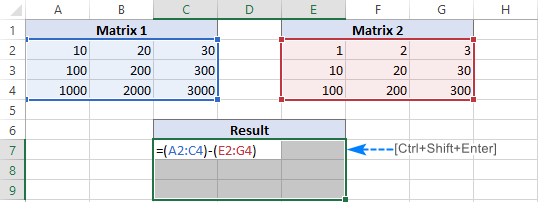The results of the subtraction will appear in the selected range. If you click on any cell in the resulting array and look at the formula bar, you will see that the formula is surrounded by {curly braces}, which is a visual indication of array formulas in Excel: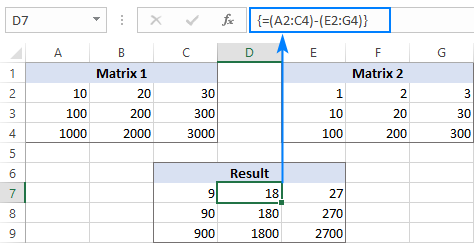If you do not like using array formulas in your worksheets, then you can insert a normal subtraction formula in the top leftmost cell and copy in rightwards and downwards to as many cells as your matrices have rows and columns.

In this example, we could put the below formula in C7 and drag it to the next 2 columns and 2 rows:

=A2-C4

Due to the use of relative cell references (without the \$ sign), the formula will adjust based on a relative position of the column and row where it is copied: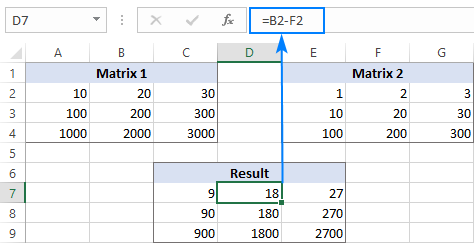## Subtract text of one cell from another cell

Here are all the observational notes using the formula in Excel
Notes :

1. The subtract methods only apply to numbers not text.
2. Now you can subtract any value in excel using the explained procedure.

Hope this article about How to use Subtraction in Excel is explanatory. Find more articles on calculating values and related Excel formulas here. If you liked our blogs, share it with your friends on Facebook. And also you can follow us on Twitter and Facebook. We would love to hear from you, do let us know how we can improve, complement or innovate our work and make it better for you. Write to us at info@exceltip.com.

Related Articles :

How to get Addition in Excel : Perform addition in Excel using SUM function or  + (plus sign) in Excel.

How to get Multiplication in Excel : Perform addition in Excel using PRODUCT function or  * (asterisk sign) in Excel

How to get Dividation in Excel : Perform addition in Excel using DIVIDE function in Excel.

How to get Quotient in Excel : Perform addition in Excel using divide option or  / in Excel.

How to get Remainder in Excel : Perform addition in Excel using MOD function in Excel.

Popular Articles :